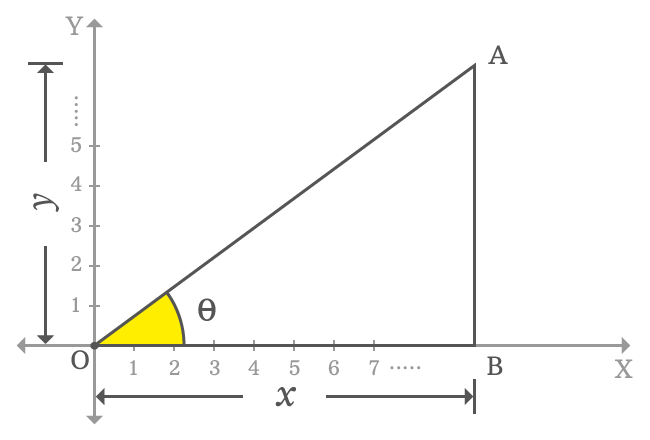# Sign of cos function in first quadrant

## Sign

$\cos{\theta} \, > \, 0$

The sign of value of cos function in first quadrant is always positive.

### Proof

$\Delta AOB$ is a right triangle in the first quadrant of the two dimensional space. The angle of the right triangle is taken as theta. The sign of cos function in first quadrant is evaluated by the ratio of lengths of adjacent side to hypotenuse. Therefore, let’s find the sign of cos function in first quadrant geometrically.In first quadrant, the $x$-axis and $y$-axis both represent positive values. So, the lengths of the adjacent side and opposite side are denoted by $x$ and $y$ respectively. Mathematically, they are written as $x > 0$ and $y > 0$.

$\cos{\theta} \,=\, \dfrac{OB}{OA}$

$\implies \cos{\theta} \,=\, \dfrac{x}{\sqrt{x^2+y^2}}$

The length of adjacent side is $x$ and it is positive. The length of opposite side is $y$ and it is also positive in this case. So, the value in the numerator is positive and the square root of sum of squares of $x$ and $y$ in denominator is also positive.

$x > 0$ and $\sqrt{x^2+y^2} > 0$. Therefore, the sign of ratio between them must be positive mathematically and it proves that the sign of the value of cos function in first quadrant is positive.

$\, \therefore \,\,\, \cos{\theta} \, > \, 0$

A best free mathematics education website that helps students, teachers and researchers.

###### Maths Topics

Learn each topic of the mathematics easily with understandable proofs and visual animation graphics.

###### Maths Problems

A math help place with list of solved problems with answers and worksheets on every concept for your practice.

Learn solutions

###### Subscribe us

You can get the latest updates from us by following to our official page of Math Doubts in one of your favourite social media sites.